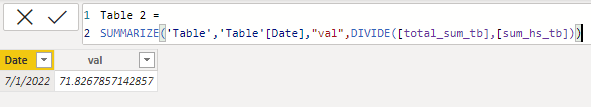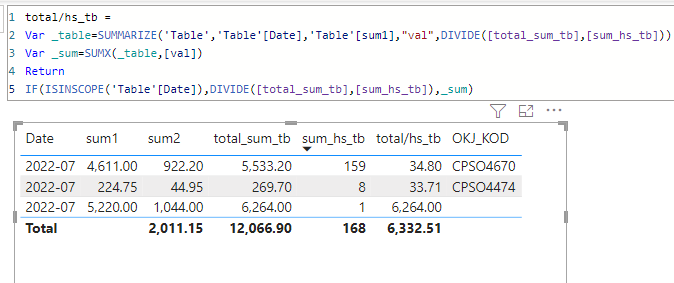cancel
Showing results for
Did you mean:Post Prodigy

## Wrong calculation if adding column in table in Power BI

Hi,

I am having question. I have situation when in one case sum of rows values in table is correct in another case when I adding new column in visual, then appears new row and sum of rows becomes incorrect. How I could fix it?6264,00+33,71+34,80 is not equal 6298,75 This comes 3 lines if I add OBJ_KOD column.

6264,00+34,80 is equal 6298.. Here getting two lines without adding OBJ_KOD column. in table

So how to resolve this issue?

``````total/hs_tb =
IF (
ISINSCOPE ( tb[OPALGA_MEN] ),
DIVIDE ( [total_sum_tb], [sum_hs_tb] ),
VAR summaryTable =
VALUES ( tb[OPALGA_MEN] ),
"@val", DIVIDE ( [total_sum_tb], [sum_hs_tb] )
)
RETURN
SUMX ( summaryTable, [@val] )
)``````

Here is a measure for a last column in visual.

1 ACCEPTED SOLUTIONCommunity Support

Hi, @Analitika

This part of your formula should be problematic.

``````VAR summaryTable =
VALUES ( tb[OPALGA_MEN] ),
"@val", DIVIDE ( [total_sum_tb], [sum_hs_tb] )
)``````

Depending on the data you provide, the dates are the same and such a result will appear.So the result of summation is not quite right.

You can try the following methods.

``````total/hs_tb =
Var _table=SUMMARIZE('Table','Table'[Date],'Table'[sum1],"val",DIVIDE([total_sum_tb],[sum_hs_tb]))
Var _sum=SUMX(_table,[val])
Return
IF(ISINSCOPE('Table'[Date]),DIVIDE([total_sum_tb],[sum_hs_tb]),_sum)``````
'Table'[sum1] This represents a column of unique values that will not be deduplication.At this time, whether you add OBJ_KOD column or not, the result will not change.

Best Regards,

Community Support Team _Charlotte

If this post helps, then please consider Accept it as the solution to help the other members find it more quickly.Community Support

Hi, @Analitika

This part of your formula should be problematic.

``````VAR summaryTable =
VALUES ( tb[OPALGA_MEN] ),
"@val", DIVIDE ( [total_sum_tb], [sum_hs_tb] )
)``````

Depending on the data you provide, the dates are the same and such a result will appear.So the result of summation is not quite right.

You can try the following methods.

``````total/hs_tb =
Var _table=SUMMARIZE('Table','Table'[Date],'Table'[sum1],"val",DIVIDE([total_sum_tb],[sum_hs_tb]))
Var _sum=SUMX(_table,[val])
Return
IF(ISINSCOPE('Table'[Date]),DIVIDE([total_sum_tb],[sum_hs_tb]),_sum)``````
'Table'[sum1] This represents a column of unique values that will not be deduplication.At this time, whether you add OBJ_KOD column or not, the result will not change.

Best Regards,

Community Support Team _Charlotte

If this post helps, then please consider Accept it as the solution to help the other members find it more quickly.